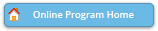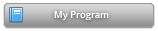#### Abstract Details

 Activity Number: 665 - Regression Methods for Longitudinal Data Type: Contributed Date/Time: Thursday, August 1, 2019 : 10:30 AM to 12:20 PM Sponsor: Section on Nonparametric Statistics Abstract #307253 Presentation Title: A Monotonic Relationship with an Ordinal Variable in Regression? How Many Degrees of Freedom Would That Use Up? Author(s): Julia Elizabeth (Kelsall) Crook* Companies: Mayo Clinic Keywords: Alzheimer's; degrees of freedom; monotonic; ordinal; regression Abstract: INTRODUCTION: In Alzheimer's disease research, many of the variables are ordinal (e.g. disease stage, Braak, Thal). One way of including an ordinal covariate in a regression is as a monotonic function. When the sample size is small we might be concerned about how many more degrees of freedom (DOF) this would use up compared to the 1 or 2 DOF that we typically devote to a variable. METHODS: The likelihood ratio test statistic for a monotonic function of an ordinal variable with k+1 categories is distributed as a weighted mixture of chi-squares on 1 to k DOF. We propose calculating k* as the weighted mean of 1 to k, with the same weights, and referring to it as the approximate effective no. of DOF. RESULTS: The value of k* depends on the relative sample sizes in each of the k+1 categories of the ordinal variable. When there are 3 categories of equal size, so that k=2, we find that k*=1.5. When the first and last categories have much higher sample sizes than those in the middle, so that the variable is almost binary, we indeed find that k* is close to 1. CONCLUSIONS: Calculation of the effective DOF can be useful when considering including monotonic terms in regression models.

Authors who are presenting talks have a * after their name.